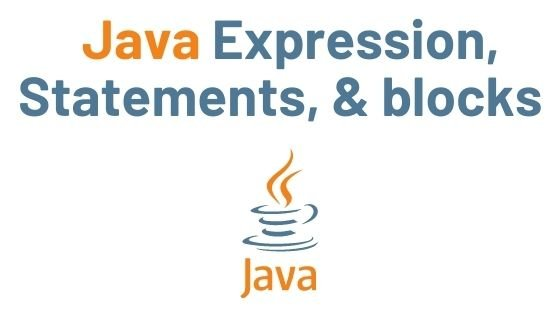## Java Expressions, Statements, and blocks | Java Tutorial

In this tutorial, you will learn about Java expressions, Java statements, the difference between expression and statement, and Java blocks.

Contents

## Java Expressions

A Java program’s work is done by expressions. An expression is a set of variables, operators, and method calls that evaluate a single value and are constructed according to the syntax of the language.

Here, value = 50 is an expression that returns an int.

## Java Statements

Java statements, like declaration and string statements, are instructions that tell the programming language what to do. Basic statements define variables and begin the execution of Java methods or blocks of other statements. Variables are assigned values using assignment statements.

• Each statement in Java is a complete unit of execution.
• We have a statement here. This statement is fully executed by multiplying integers 5 and 5 and then assigning the result to the variable value.

We have an expression 5 * 5 in the preceding statement. Expressions are part of statements in Java.

## Expression statements

A semicolon is followed by an expression in an expression statement. The expression statements I = 0; I = I + 1; and printf(“Developersdome!”); are all expression statements.

• In the preceding example, the expression value = 5 is used. We’ve turned the expression into a statement (value = 5;) by adding a semicolon (;).

## Declaration Statements

• Declarative statements are used to declare variables in Java.
• A declaration statement is a type of Java statement that is used to declare a variable by naming it and specifying its data type. Some declaration statements are shown below. In Java programming, a variable is a container that holds values used in a Java program.

The above statement declares a variable value with a value of 5.

lambda expression j,lambda expression java,lambda expression javalambda expression java,ava,lambda expression java,lambda expression java, lambda expression j,lambda expression java,lambda expression javalambda expression java,ava,lambda expression java,lambda expression java , lambda expression j,lambda expression java,lambda expression javalambda expression java,ava,lambda expression java,lambda expression java

## Java Blocks

In Java, a block is a collection of one or more statements enclosed by braces. An opening brace () and a closing brace () start and end a block. You can code one or more statements between the opening and closing braces.

```class Main {
public static void main(String[] args) {

String name = "DeveloperDome";

if (name == "DeveloperDome") { // block starting
System.out.print("hello");
System.out.print("Android development!");
} // block ending
}
}```

Output:

You might also like:

Java Input and Output | Java Tutorial

Operators in Java | Java Tutorial

Java Data Types | Primitive and Non-Primitive Data Types

We hope that this article will assist you in understanding all about Java Expression, Statements, and blocks. We have concentrated on making a basic, meaningful, and easy-to-learn guide to the concepts. Still, if you have any problems regarding this, please post them in the comment section, we will be glad to assist you.

java regular expression, java regular expression, java regular expression, java regular expression, java regular expression, java regular expression, java regular expression, java regular expression, java regular expression java regular expression, java regular expression, java regular expression, java regular expression, java regular expression, java regular expression, java regular expression, java regular expression, java regular expression, java regular expression java regular expression

Java Expressions, Statements, and blocks, Java Expressions, Statements, and blocks, Java Expressions, Statements, and blocks, Java Expressions, Statements, and blocks, Java Expressions, Statements, and blocks, Java Expressions, Statements, and blocks